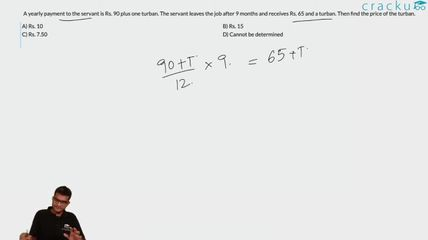Question 59

# A yearly payment to the servant is Rs. 90 plus one turban. The servant leaves the job after 9 months and receives Rs. 65 and a turban. Then find the price of the turban.

Solution

Let's say price of turban is x.

So total price for 12 months will be = $$90+x$$

total price for 9 months = $$\frac{(90+x) \times 9}{12} = (65+x)$$

By solving above equation, we will get value of x= 10.

### View Video Solution